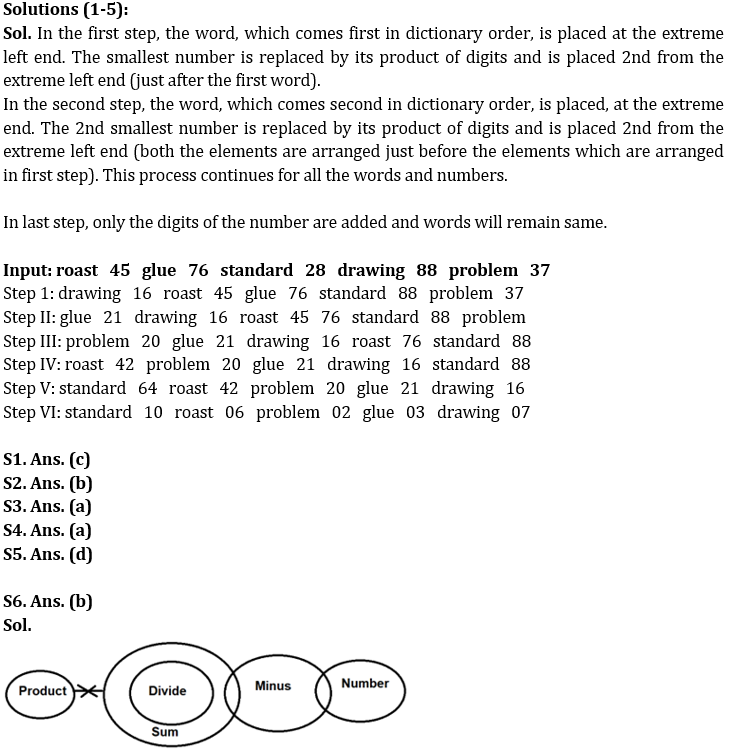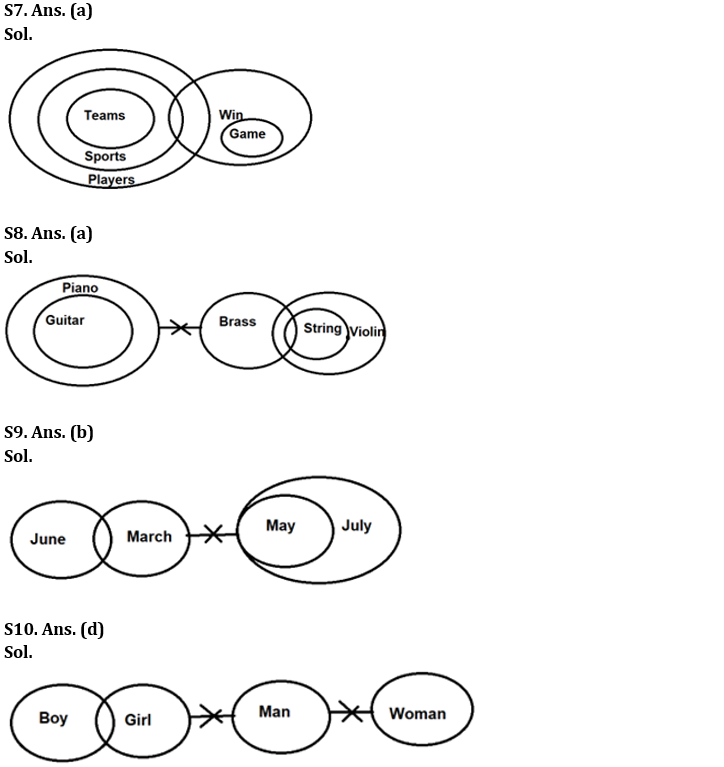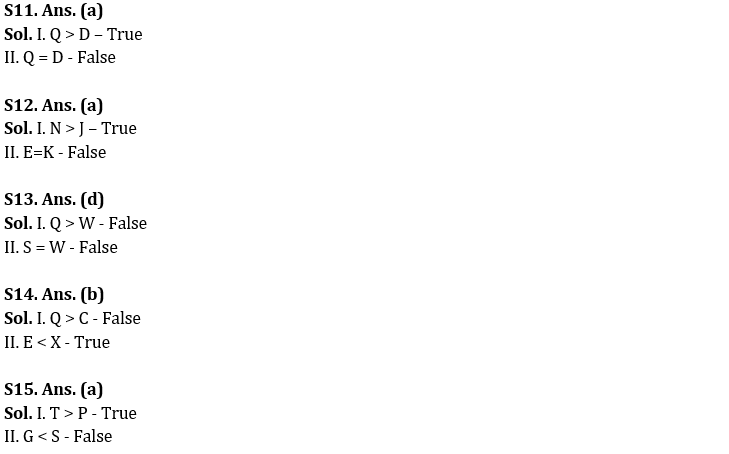Latest Banking jobs   »

# Reasoning Ability Quiz For IDBI AM/Executive 2022- 1st July

Directions (1-5): When a word and number arrangement machine is given an input line of words and numbers, it arranges them following a particular rule. The following is an illustration of input and rearrangement.

Input: naughty 46 dynamic 49 provide 38 advance 78 minus 65
Step 1: advance 24 naughty 46 dynamic 49 provide 78 minus 65
Step II: dynamic 24 advance 24 naughty 49 provide 78 minus 65
Step III: minus 36 dynamic 24 advance 24 naughty provide 78 65
Step IV: naughty 30 minus 36 dynamic 24 advance 24 provide 78
Step V: provide 56 naughty 30 minus 36 dynamic 24 advance 24
Step VI: provide 11 naughty 03 minus 09 dynamic 06 advance 06
And step VI is the last step of the rearrangement. As per the rules followed in the above step, rearrange the given input and answer the related questions.

Input: roast 45 glue 76 standard 28 drawing 88 problem 37

Q1. Which of the following is step V of the given input after the rearrangement?
(a) roast 42 problem 20 glue 21 drawing 16 standard 88
(b) standard 10 roast 06 problem 02 glue 03 drawing 07
(c) standard 64 roast 42 problem 20 glue 21 drawing 16
(d) glue 21 drawing 16 roast 45 76 standard 88 problem
(e) None of these

Q2. In which step of the given input, ‘64 roast 42 problem’ will appear in the same sequence after the rearrangement?
(a) Step III
(b) Step V
(c) Step VI
(d) Step IV
(e) None of these

Q3. What is the difference between 5th number from the right end and 4th number from the left end in step IV of the given input after the rearrangement?
(a) 26
(b) 25
(c) 21
(d) 28
(e) 27

Q4. Which of the following element is 5th from the left end in step II of the given input?
(a) Roast
(b) 16
(c) Glue
(d) 45
(e) Drawing

Q5. Which of the following element does not appear in step V of the given input?
(a) 42
(b) 20
(c) Glue
(d) 88
(e) Drawing

Directions (6-10): In the questions below, three statements are given followed by the conclusions. You have to take the given statements to be true even if they seem to be at variance with commonly known facts. Read all the conclusions and then decide which of the given conclusions logically follows from the given statements disregarding commonly known facts.

Q6. Statements: Only Sum are Divide. Some Minus are Sum. Only a few Minus are Number. No Sum are Product.
Conclusions: I. A few Number being Divide is a possibility
II. Some Minus are not Product
(a) If only conclusion I follows
(b) If only conclusion II follows
(c) If either conclusion I or conclusion II follows
(d) If both conclusions I and II follow
(e) If neither conclusion I nor conclusion II follows

Q7. Statements: All Teams are Sports. All Sports are Players. Only Win are Game. A few Sports are Win.
Conclusions: I. At least Some Sport are Team
II. At least Some Teams are Win
(a) If only conclusion I follows
(b) If only conclusion II follows
(c) If either conclusion I or conclusion II follows
(d) If both conclusions I and II follow
(e) If neither conclusion I nor conclusion II follows

Q8. Statements: Only Piano are Guitar. No Piano is Brass. Only a few Brass are Strings. All Strings are Violin.
Conclusions: I. A few Strings being Guitar is not a possibility
II. No Brass is Violin
(a) If only conclusion I follows
(b) If only conclusion II follows
(c) If either conclusion I or conclusion II follows
(d) If both conclusions I and II follow
(e) If neither conclusion I nor conclusion II follows

Q9. Statements: No March is May. Only a few June is March. All May is July.
Conclusions: I. Some March is July.
II. All March being June is a possibility.
(a) If only conclusion I follows
(b) If only conclusion II follows
(c) If either conclusion I or conclusion II follows
(d) If both conclusions I and II follow
(e) If neither conclusion I nor conclusion II follows

Q10. Statements: Only a few boy is girl. No girl is man. No man is woman.
Conclusions: I. Some woman being girl is a possibility
II. A few boy can never be man
(a) If only conclusion I follows
(b) If only conclusion II follows
(c) If either conclusion I or conclusion II follows
(d) If both conclusions I and II follow
(e) If neither conclusion I nor conclusion II follows

Directions (11-15): In the following questions assuming the given statement to be true and find which of the conclusion(s) among given conclusions is/are definitely true and then give your answers accordingly.

Q11. Statements: T < A < B = C ≤ D = F, F < O ≤ L = Q
Conclusions: I. Q > D
II. Q = D
(a) Only I is true
(b) Only II is true
(c) Either I or II is true
(d) Neither I nor II is true
(e) Both I and II are true

Q12. Statements: L > N = T = O, O > E = F ≥ G ≥ K > J
Conclusions: I. N > J
II. E=K
(a) Only I is true
(b) Only II is true
(c) Either I or II is true
(d) Neither I nor II is true
(e) Both I and II are true

Q13. Statements: X< Q ≥ S=R, R=Y ≥ U=V≥ W
Conclusions: I. Q > W
II. S = W
(a) Only I is true
(b) Only II is true
(c) Either I or II is true
(d) Neither I nor II is true
(e) Both I and II are true

Q14. Statements: Q ≥ P < X; P ≥ B < C; E = S < B
Conclusions: I. Q > C
II. E < X
(a) Only I is true
(b) Only II is true
(c) Either I or II is true
(d) Neither I nor II is true
(e) Both I and II are true

Q15. Statements: T = S > V ≥ M; C ≤ M; G >P < C
Conclusions: I. T > P
II. G < S
(a) Only I is true
(b) Only II is true
(c) Either I or II is true
(d) Neither I nor II is true
(e) Both I and II are true

Solutions#### Congratulations!Incorrect details? Fill the form again here

•Reasoning Ability Quiz For IBPS RRB PO C...
•Reasoning Ability Quiz For IBPS Clerk Pr...
•Reasoning Ability Quiz For IBPS RRB PO C...
•Reasoning Ability Quiz For IBPS RRB PO C...
•Reasoning Ability Quiz For IBPS RRB PO C...
•Reasoning Ability Quiz For IBPS Clerk Pr...